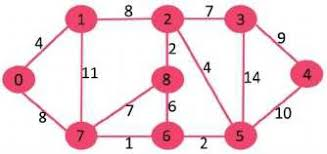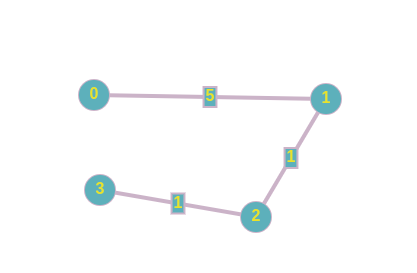0

# Path more than distance K

Difficulty: EASY
Avg. time to solve
20 min
Success Rate
80%

Problem Statement
Suggest Edit

#### You are given an undirected graph, a source vertex in the graph which will always be 0 (starting vertex), and a number k. Your task is to find if there is a simple path, of path length greater than k,(without any cycle) starting from the given source vertex and ending at any other vertex. A simple path is that path in a graph that does not form a cycle.

##### Note:
``````Assume that the source vertex to always be  0.
``````
##### For Example:
``````Here V = 9, E = 14 and K = 60
``````Output: YES Explanation: There exists a simple path 0 -> 7 -> 1-> 2 -> 3 -> 4 -> 5-> 6 -> 8 Which has a total distance of 61 units which is more than 60.

##### Input format:
``````The first line contains space-separated integers 'V', ’E’ and ‘K’ where ‘V’ is the number of vertices in the graph, ‘E’ the number of edges
while ‘K’ denotes the sum of the weights in the simple path which should be greater than ‘K’

Then ‘E’ lines follow. Each line contains 3 space-separated integers denoting the values
where the first value is vertex V1, next is vertex V2 and the last value is the weight (W) of the edge between vertices V1 and V2, respectively.
``````
##### Output format:
``````For the given graph, set of edges, vertices and value ‘K’, print ‘YES’ if there exists a simple path with the sum of weights greater than ‘K’ and ‘NO’ if there is no such path.

Output for each test case will be printed in a separate line.
``````
##### Note:
``````You do not need to print anything. It has already been taken care of. Just implement the given function.
``````
##### Constraints:
``````2 ≤ V ≤ 10
1 ≤ E ≤ 20
1 ≤ K ≤ 100

where ‘V’ is the number of vertices in the graph, ‘E’ the number of edges while ‘K’ denotes the sum of the weights in the simple path which should be greater than ‘K’

Time limit: 1 second
``````
##### Sample Input 1:
``````4 3 8
0 1 5
1 2 1
2 3 1
``````
##### Sample Output 1:
``````NO
``````
##### Explanation for sample 1:
``````The graph corresponding to the first test case is -
````````````There exists no path which has a distance greater than equal to 8.
``````
##### Sample Input 2:
``````9 14 60
0 1 4
0 7 8
1 2 8
1 7 11
2 3 7
2 5 4
2 8 2
3 4 9
3 5 14
4 5 10
5 6 2
6 7 1
6 8 6
7 8 7
``````
##### Sample Output 2:
``````YES
``````Want to solve this problem? Login now to get access to solve the problems